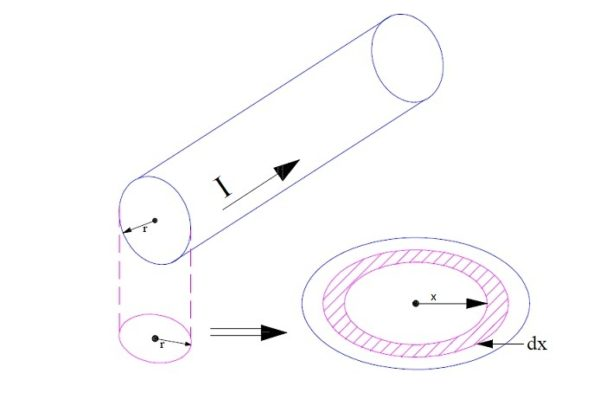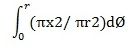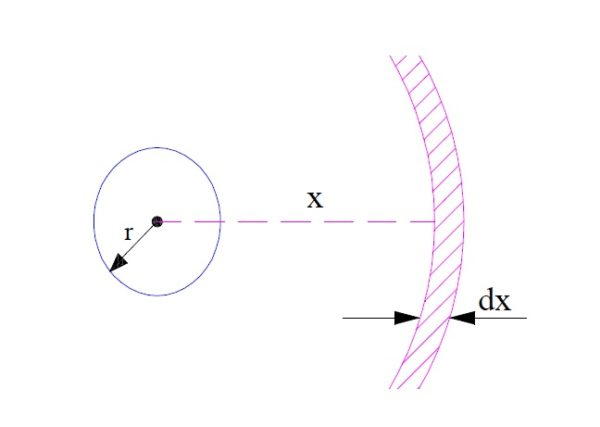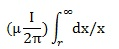# Inductance of Single Conductor of Transmission Line

Inductance is the property of a conductor due to which it opposes a sudden change in current through it and a basic electrical parameter like resistance and capacitance. Inductance is defined as the total flux linkage per unit current. To analyse the electrical behavior of circuit, it is very important to have knowledge of all electrical parameter of the related circuit. In this post, we will calculate the inductance of Single Conductor of Transmission Line.

## Inductance of Single Conductor of Transmission Line

Let us consider a single conductor in which current I is flowing as shown in figure below.To calculate the inductance, we need to first calculate the flux linkage of conductor. The conductor will produce a magnetic flux in its surrounding due its own current ‘I’. The flux linking of conductor thus may be divided into two category.

• Flux linkage due to internal flux
• Flux linkage due to external flux

We will calculate each type of flux linkage of conductor and then will add up them to get the total flux linkage. Once total flux linkage is calculated, inductance will be equal to total flux linkage divided by current ‘I’.

### Flux Linkage due to Internal Flux:

Let us assume as a conductor of radius ‘r’. Current I is supposed to be uniformly distributed over the entire cross section of conductor.

Consider a differential strip of thickness dx at a distance of x from the centre of conductor as shown in figure above. Let the current flowing through the conductor of radius ‘x’ be Ix then

Ix = I (πx2/ πr2) = I (x2/ r2)

Magnetic Field Intensity due to this current

Hx =  Ix/2πx

= Ix/(2πr2) AT/m

Assuming permeability of conductor to be µ, magnetic flux density at the considered point x,

Bx = µHx

= µIx/(2πr2) Tesla

Now, flux through assumed cylindrical shell of thickness dx and axial length 1 is given as

dØ = Bxdx = µIxdx/(2πr2) Weber

This flux dØ only links with current Ix within the considered area, therefore total flux linkage of single conductor due to internal flux can be found by integrating from 0 to r.

Øint == µI/8π

### Flux Linkage due to External Flux:

Now we will calculate the flux linkage of conductor due to external flux. Mind that this external flux is also created by the own current of conductor and extends from the surface to infinity.Let us again consider a cylindrical shell of radius ‘x’ and thickness dx as shown above. The magnetic field intensity at the surface of cylindrical shell

H = I/2πx (current I is carried by conductor)

So,

Magnetic Flux Density at surface B = µH

= µI/2πx

Therefore, flux through the considered cylindrical shell

dØ = Bdx

= µIdx/2πx Wb

This flux dØ links all the current I passing through the conductor, hence total flux linking of single conductor due to external flux is given as

ØextHence, ØextTherefore, total flux linkage of conductor

ψ = Øint + Øext

Hence, Inductance of Single Conductor L = ψ / I

This site uses Akismet to reduce spam. Learn how your comment data is processed.